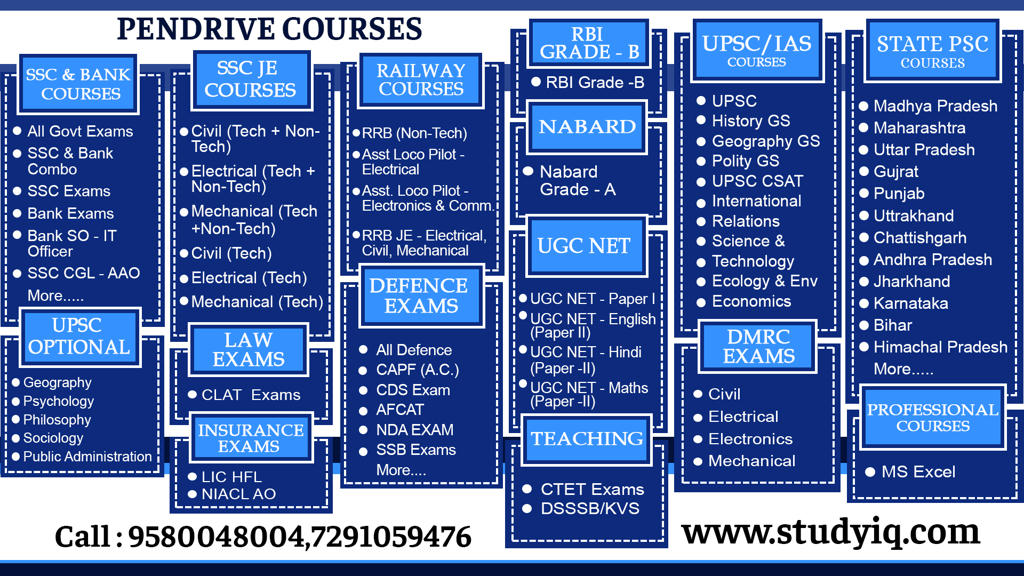# Profit and Loss Questions – Part 1 – Maths & Reasoning | PDF DownloadQ1. The ratio of cost price and selling price of an article is 8:9 the profit percentage is

Q2. A shopkeeper sells a commodity at rupees 2149 and earned 16.66 % profit find the cost price of the commodity is

Q3. When an object is ₹4214 rupees loss is 12.5 % find the cost price of the object

Q4. The cost price of 15 articles is same as the selling price of 10 articles the profit percentage

Q5. The cost price of 10 articles is equal to the selling price of 9 articles find the profit percent

Q6. Selling price of 9 objects is equal to the cost price of 12 objects find the profit or loss percentage

Q7. The loss percent incurd when an object is sold at 436 Rupees is equal to the profit percent which is gained if the object is sold at rupees 464 find the cost price of that object

Q8. By selling an object for rupees 900 a man earns double the profit of what he would have lost had he sold it 4 rupees 450 if he sells it at a profit of 20% find its selling price

Q9. after selling an article for Rs 1060 shopkeeper earns 20% more profit than the loss incurred after selling the article at rupees 950

Q10. A shopkeeper sells an article for Rs 78 and earns double the profit that he would have earned had he sold it for rupees 69 what is its cost price

Q11. A man buys pencils at the rate of 6 for Rs 5 and sells them at 5 for Rs 6 . his profit percent is

Q12. A man buys lemons at the rate of 11 for rupees 10 and sells them at 10 for 11 what is his profit or loss percent

Q13. A man buys lemons at the rate of 11 for rupees 10 and same number at 9 for Rs 10 the whole lot was sold at 1 Rupee per toffee profit and loss is the whole transaction was

Q14. A shopkeeper buys some lemons at 2 for rs 1 and same number of lemons at 1 for rs 2 he sells them at 3 for rs 3 what is his gain or loss percent in this

Q15. A man buys lemons at the rate of 11 for rupees 10 and sells them at 10 for 11 , to earn 42rs profit find the number of lemon which he buy

Q16. A man buys lemons at the rate of 8 for rupees 34 and sells them at 12 for 57 RS to earn 45rs profit find the number of orange which he buy

Q17. Nitin bought some oranges with a price of 40 per dozen and sold as many oranges of ₹30 per dozen and received a loss of 480, then find the number in the number of orange in dozen bought by him.

Q18. A fruit seller buys 700 oranges at a rate of 100 oranges of 500 ₹ 500 and other varieties buy 500 oranges at the rate of 100 ₹ 700 orange and sell them ₹ 84 per dozen, then calculate the profit percentage

Q19. By selling 33 M of cloth A shopkeeper earns profit equivalent to the cost price of 11 M. find his profit percent

Q20. Selling 33 minutes cloth A shopkeeper earns profit equivalent to selling price of 11 metre find his profit percent

Q21. By selling 33 metre cloth A losses money equivalent to selling price of 11 metre find his loss percentage

Q22. Mohan bought 20 dining tables for Rs 32000 and sold them at a profit equal to the selling price of 4 dining tables. The selling price of each dining table is:
1. 2000
2. 2500
3. 1500
4. 1600

Q23. Mohan bought 16 dining tables for Rs 18000 and sold them at a profit equal to the selling price of 4 dining tables. The selling price of each dining table is:
1. 1500
2. 750
3. 1000
4. 2000